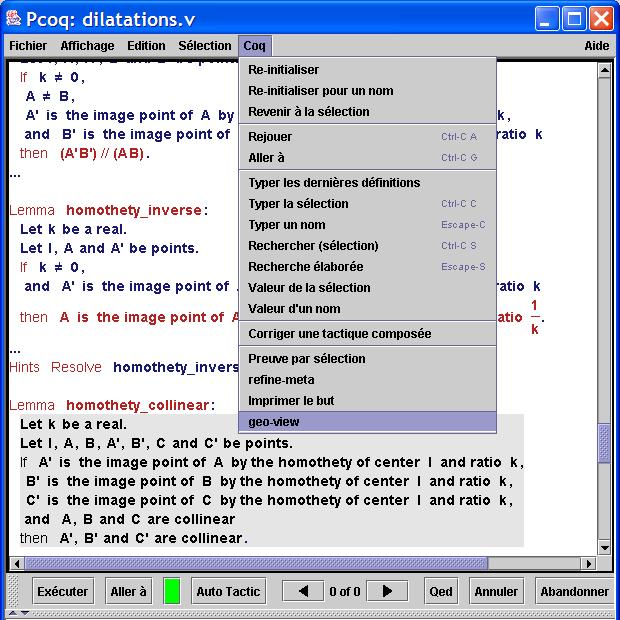Cette page en Français

 GeoView

GeoView is a tool that combines the Coq theorem prover with the dynamic geometry drawing tool GeoplanJ developed at CNAM.
GeoView is an extension of the user-interface Pcoq and is dedicated to the visualization of formal statements of plane geometrical theorems.

The statements of geometrical theorems and their proofs are constructed and verified with Coq theorem prover.
A dynamic figure is automatically associated to mathematical formulas to show the meaning of these statements.
The figures generated with GeoView can be integrated as interactive and dynamic figures in HTML files using the GeoplanJ applet.

Here is an example of a statement dealing with homothety and collinearity (you can see other examples here).And here is the dynamic figure generated by GeoView :

You can grab the free points (in green) by pointing with the mouse and move them by dragging.
By double-clicking on the figure, you obtain a menu to create new objects (points, lines ...)
The real number k is displayed as a point on a straight line. You can change the value of k by moving this point.

GeoView is written in Java and has been integrated in the graphical interface Pcoq developed and maintained in the Lemme team at INRIA Sophia-Antipolis.